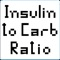# Insulin To Carb (I:C) Ratio Calculator

価格 ｼﾞｬﾝﾙ サイズ このアプリは現在ストアで閲覧することができません。 無料 ダウンロードヘルスケア／フィットネス 67.8KB Pancreum ｼﾞｬﾝﾙ別：---総合：--- 2010-05-18 10:07:44 評価が取得できませんでした。 iOS 3.1.3以降が必要です。 iPhone、iPad および iPod touch 対応。 2022-08-13　このアプリは現在ストアから情報が取得できません。削除された可能性があります。
InsToCarb calculates your Insulin-to-Carbohydrate ratio (also known as I:C Ratio, Carbohydrate Factor or Carbohydrate-To-Insulin Ratio). It is based on inputs such as an individual's weight (kg and lbs), height (cm and ft/in), age and gender. It calculates the BMI (Body Mass Index) based on the weight and height, makes minor statistical adjustments to it for age and gender, calculates the TDD (Total Daily Dose) of insulin based on the adjusted BMI, and finally calculates the ratio.

BMI (Body Mass Index) Calculation:
The standard BMI is calculated from the weight (in kg) and the height (in m).

Statistical research shows that females tend to have an average BMI that is 5 - 10% higher than men. Researchers believe this should not affect the total daily dose of insulin for females and their weight classification bracket should be adjusted to compensate for this minor difference.

Research shows that individuals with age ranging from 2 to 19 years of age tend to have proportionately lower BMI than individuals 20 or older. Due to this statistical fact, researchers believe this should be taken into consideration when calculating the total daily dose of insulin for young individuals, and their percentile classification bracket should be adjusted to compensate for that difference.

TDD (Total Daily Dose) of Insulin Calculation and Adjustment for BMI:
The TDD calculation is based on an individual’s weight (in kg) multiplied by a factor related to the BMI. There are 4 factors/brackets used: Frail/Thin/Lean (smallest factor), Healthy, Overweight and Obese (largest factor).

The I:C Ratio is needed as parameter used in calculating a corrective or meal insulin bolus. The calculated value is expressed in grams per unit of insulin.

The I:C Ratio defines how many grams of carbohydrate, after digestion and dispersal into the extra-cellular space (ECS), will be transported per unit of insulin into insulin sensitive cells for metabolism or energy storage. The number of grams of carbohydrate transferred across cell membranes per unit measures insulin efficiency and is directly related to an individual's insulin sensitivity, so an individual's I:C ratio is directly proportional to their insulin sensitivity.

Carbohydrate intake per day can be divided by the carbohydrate boluses, which is proportional to TDD. It is based on the average ratio for a large group of well-controlled individuals with Type 1 diabetes.

Other factors taken into consideration were:

(1) An individual's ratio is directly proportional to their IS. If individuals of different weights both consume 50 grams of carbohydrate but have the same IS, they will require identical carbohydrate boluses to cover these carbohydrates.
(2) An individual's relative IS (rIS) in Type 1 diabetes can be quickly estimated as the ratio between a TDD for someone’s weight with an average IS and compare this to one’s own TDD.
(3) A constant that represents an average sensitivity to insulin is used to calculate the expected TDD for this person if the IS were average.
(4) Once a mean actual ratio value is known for a large group of individuals in good control with a minimum of hypoglycemia, any other individual's ratio can be closely approximated by multiplying this mean ratio times the individual's rIS.
(5) Used a mean actual ratio of 10.8 g/u.

Note: This formula is based on carbohydrate counts that are likely undercounted by most pump wearers, so an individual who counts carbohydrates precisely may need to raise their ratio number calculated here.

Use this calculator only on advice from your physician. Your physician will provide you with the parameter values needed. This method for calculating the I:C Ratio is not suitable for everyone.

1. The calculated value now changes gradually over its range, as some users didn't understand the fact that the calculated results previously only changed in 4 steps, based on their calculated BMI bracket.
2. Touching the "Reset" button reloads the default values, which are just example values. Users must enter their own data after that.
3. This App does not replace a doctor, as some users seem to believe. Users should still visit their endocrinologist.
• 現在ランキング圏外です。ブログパーツ第二弾を公開しました！ホームページでアプリの順位・価格・周辺ランキングをご紹介頂けます。
ブログパーツ第2弾！

アプリの周辺ランキングを表示するブログパーツです。価格・順位共に自動で最新情報に更新されるのでアプリの状態チェックにも最適です。
ランキング圏外の場合でも周辺ランキングの代わりに説明文を表示にするので安心です。

サンプルが気に入りましたら、下に表示されたHTMLタグをそのままページに貼り付けることでご利用頂けます。ただし、一般公開されているページでご使用頂かないと表示されませんのでご注意ください。

オススメ！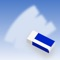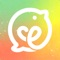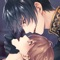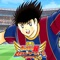お得情報ピックアップ！35%値引中50%値引中66%値引中75%値引中50%値引中Now loading...